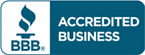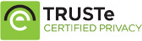# Solution manual for Introductory Econometrics A Modern Approach 4th Edition by Jeffrey M. Wooldridge

\$30.00

Introductory Econometrics A Modern Approach 4th Edition by Jeffrey M. Wooldridge Answers to questions

Full Chapters are included## Solution manual for Introductory Econometrics A Modern Approach 4th Edition by Jeffrey M. Wooldridge

Table of Contents Solution manual for Introductory Econometrics A Modern Approach 4th Edition by Jeffrey M. Wooldridge

1. The Nature of Econometrics and Economic Data.
PART 1: REGRESSION ANALYSIS WITH CROSS-SECTIONAL DATA.
2. The Simple Regression Model.
3. Multiple Regression Analysis: Estimation.
4. Multiple Regression Analysis: Inference.
5. Multiple Regression Analysis: OLS Asymptotics.
6. Multiple Regression Analysis: Further Issues.
7. Multiple Regression Analysis with Qualitative Information: Binary (or Dummy) Variables.
8. Heteroskedasticity.
9. More on Specification and Data Problems.
PART 2: REGRESSION ANALYSIS WITH TIME SERIES DATA.
10. Basic Regression Analysis with Time Series Data.
11. Further Issues in Using OLS with Time Series Data.
12. Serial Correlation and Heteroskedasticity in Time Series Regressions.
PART 3: ADVANCED TOPICS.
13. Pooling Cross Sections across Time: Simple Panel Data Methods.
14. Advanced Panel Data Methods.
15. Instrumental Variables Estimation and Two Stage Least Squares.
16. Simultaneous Equations Models.
17. Limited Dependent Variable Models and Sample Selection Corrections.
18. Advanced Time Series Topics.
19. Carrying out an Empirical Project.
APPENDICES.
Appendix A Basic Mathematical Tools.
Appendix B Fundamentals of Probability.
Appendix C Fundamentals of Mathematical Statistics.
Appendix D Summary of Matrix Algebra.
Appendix E The Linear Regression Model in Matrix Form.
Appendix F Answers to Chapter Questions.
Appendix G Statistical Tables.
References.
Glossary.
Index.

## Reviews

There are no reviews yet.